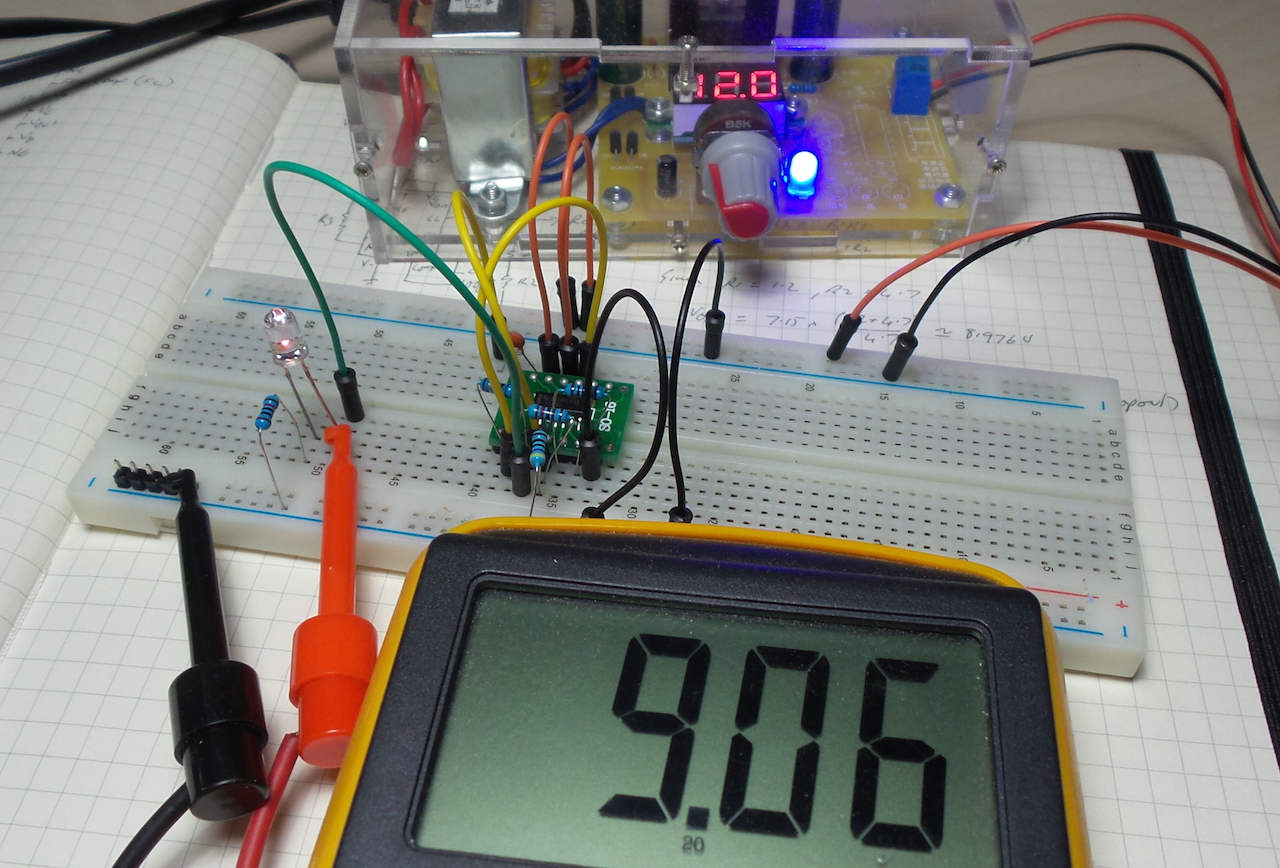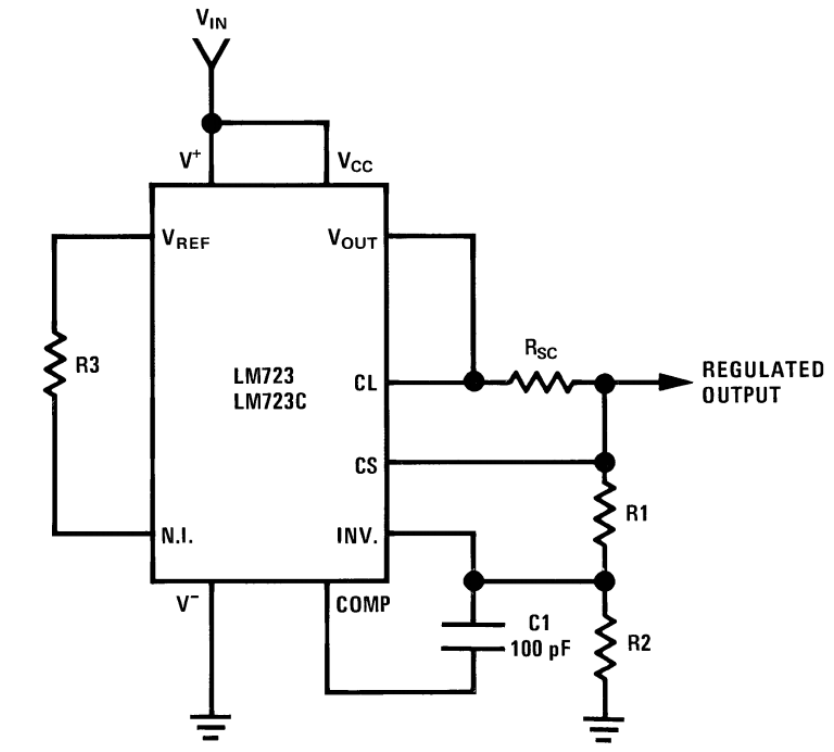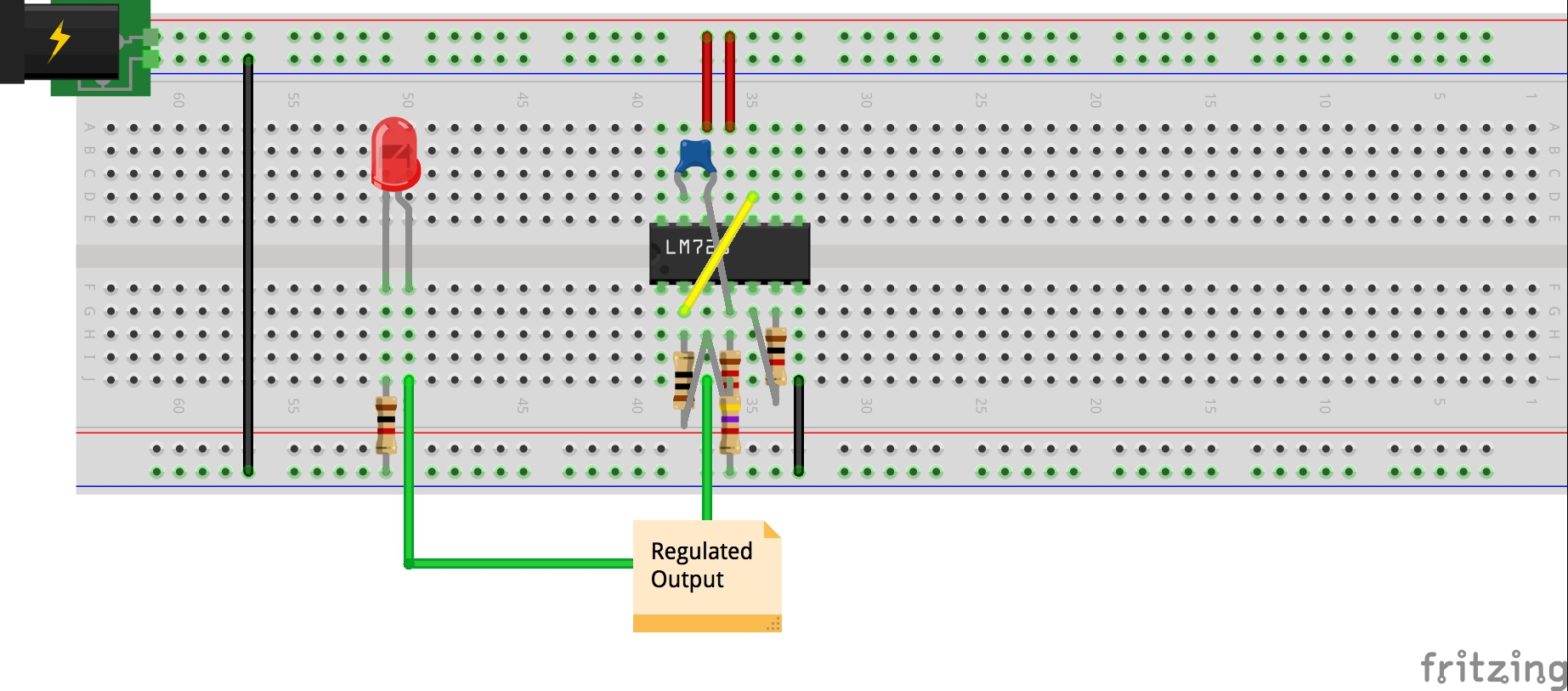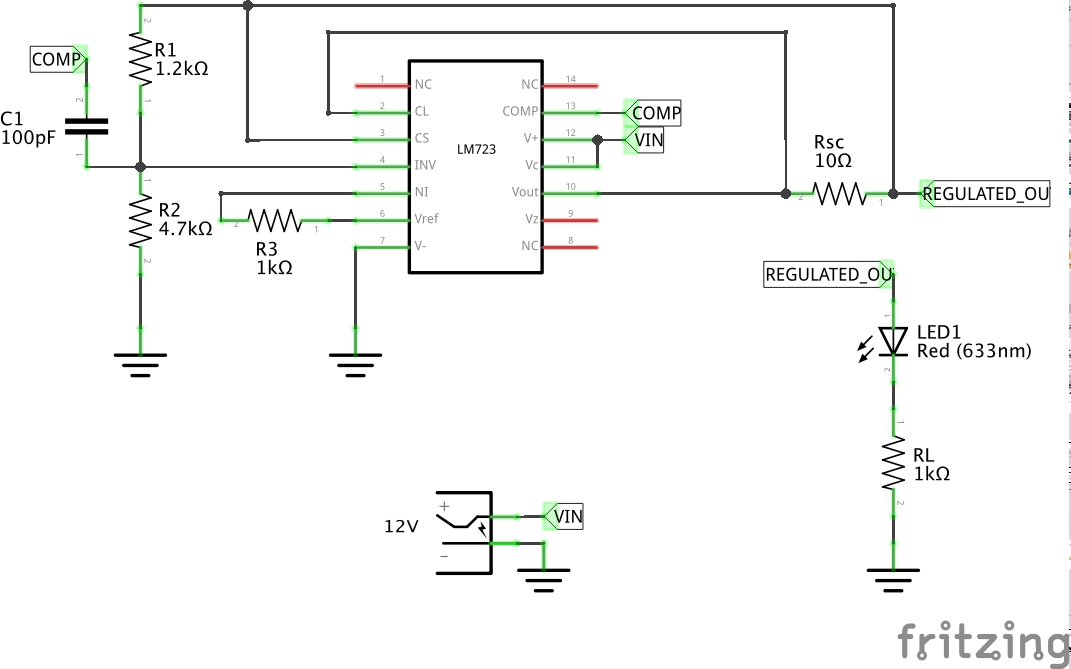#384 LM723/HighVoltageRegulator

Playing with the basic high voltage output circuit for the LM723 voltage regulator.The LM723 voltage regulator is a classic IC designed by Bob Widlar. Although not recommended or particularly useful for modern designs, it is widely discussed and documented, so makes an excellent learning tool.

This little project is a straight-forward build of the reference circuit for high voltage regulation.

Some great resources for the LM723 are:

See also LEAP#383 LM723/LowVoltageRegulator for more background and resources for the LM723.

Basic High Voltage RegulatorOutput Voltage Set

A resistor divider (R1, R2) is used to set the target voltage as a multiple of the reference voltage. This is what is meant by “high voltage regulator” - the output voltage will be greater than the reference voltage.

I’m using R1=1.2kΩ, and R2=4.7kΩ, so assuming Vref=7.15V, the expected output voltage is 8.976V

The reference voltage is fed to the error amplifier via R3. For minimum temperature drift, R3 = R1 * R2/(R1+R2) = 960Ω, say use 1kΩ

Actual performance: I’m reading a regulated output of 9.06V, slightly over the predicted value. I measure the actual R1 and R2 values and they are accurate within 10Ω. But I measure the Vref output of the LM723 at 7.26V. At this value, the expected output is closer at 9.1V.

Current Limit

Adding current limiting control is optional. It is achieved with a series sense resistor Rsc on the output, used to bridged the current sense and current limit pins, which are effectively the base and emitter pins of a current limiting transistor in the LM723.

When the current through Rsc produces a voltage drop of over about 0.5V, the current limiter starts throttling the output control transistor.

Assuming a limiting drop of 0.5V and a target current limit of 50mA, Rsc = Vbe/Ilimit = 10Ω

I haven’t tested the effectiveness of the current limit yet.

I have an LED and 1kΩ current limiting resistor as a dummy load.

Supply Rail

The 723 requires a relatively high input voltage of between 9.4 and 40 volts (it was designed before “low dropout” was a thing). The main constraint on the minimum voltage is the need to generate the 7.15V reference voltage.

I started running the circuit at 12V. As I reduce the supply voltage, it starts to lose regulation at about 10.6V.

Construction